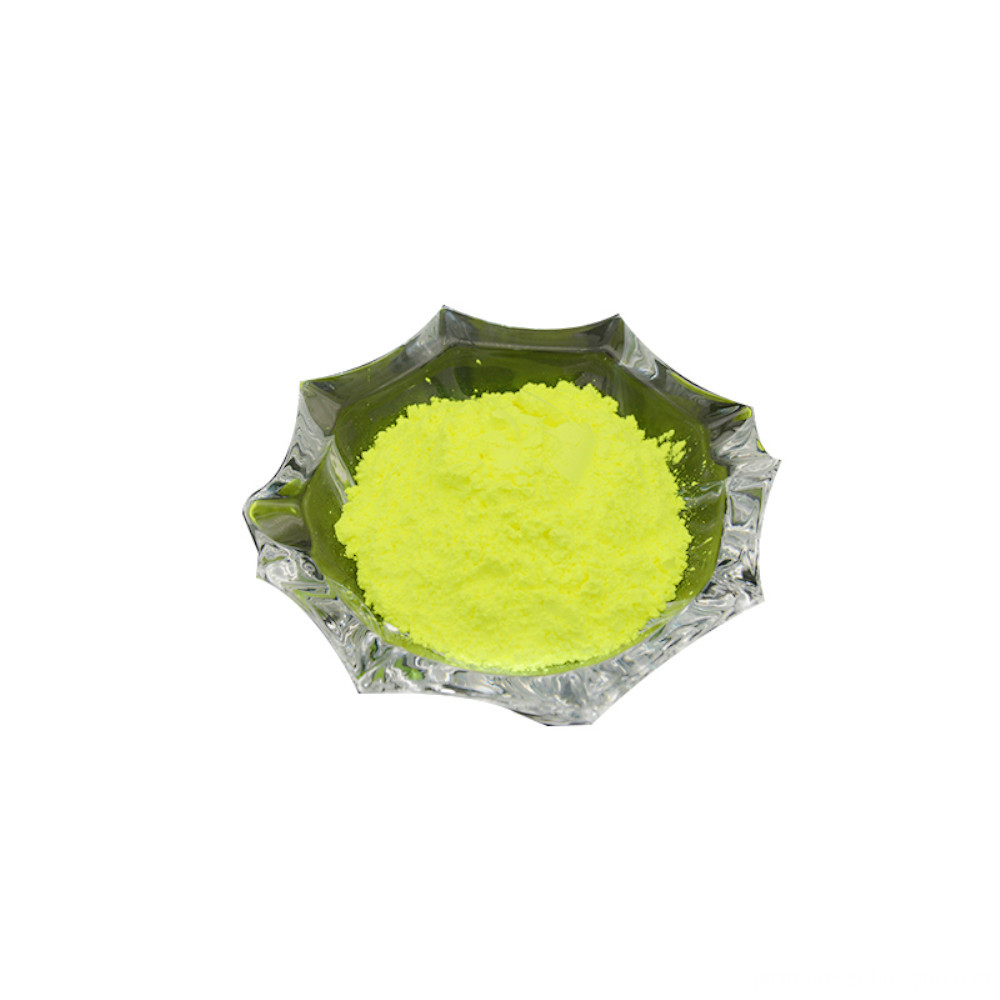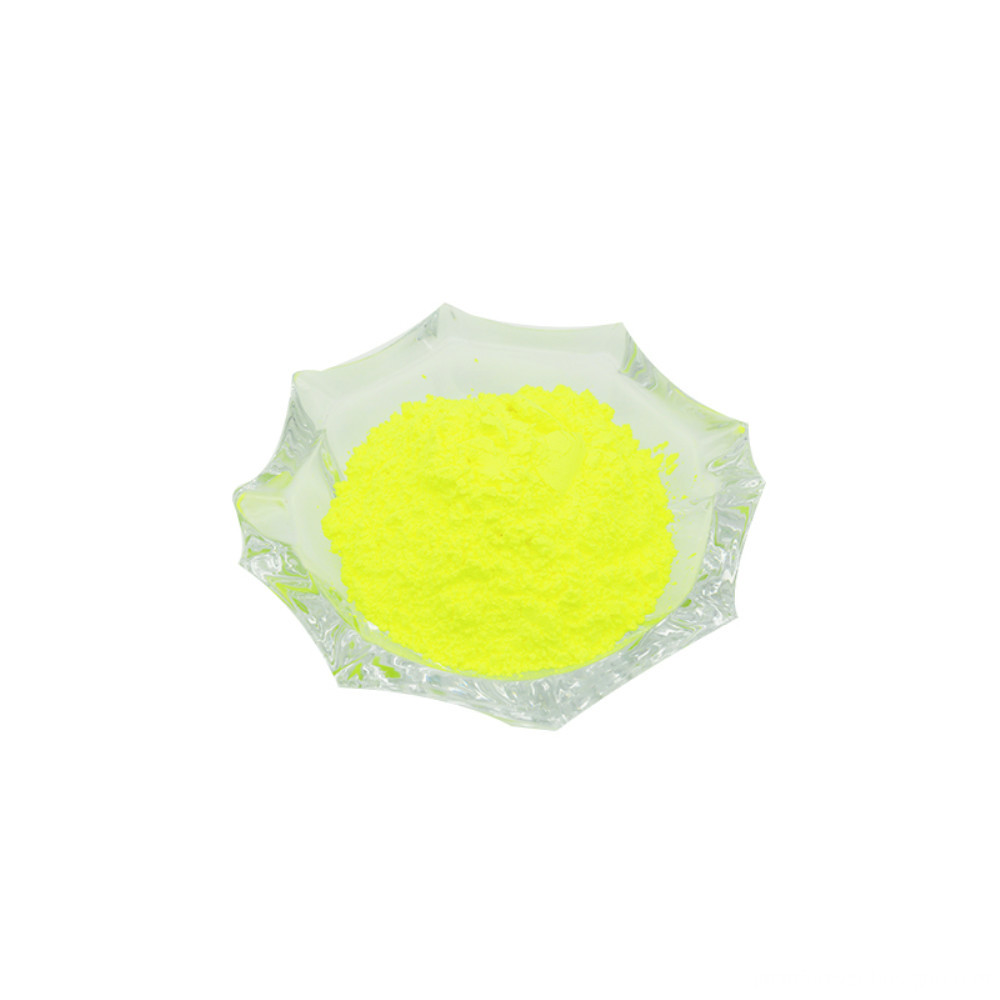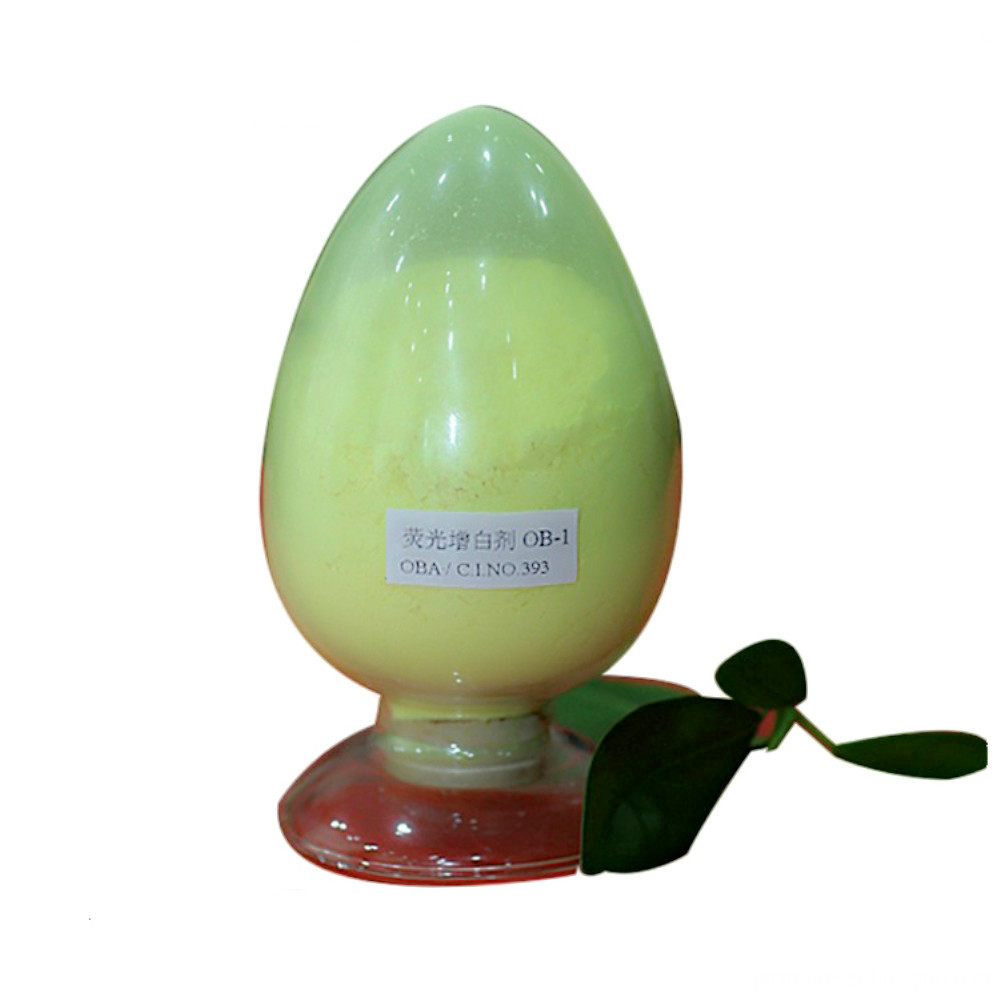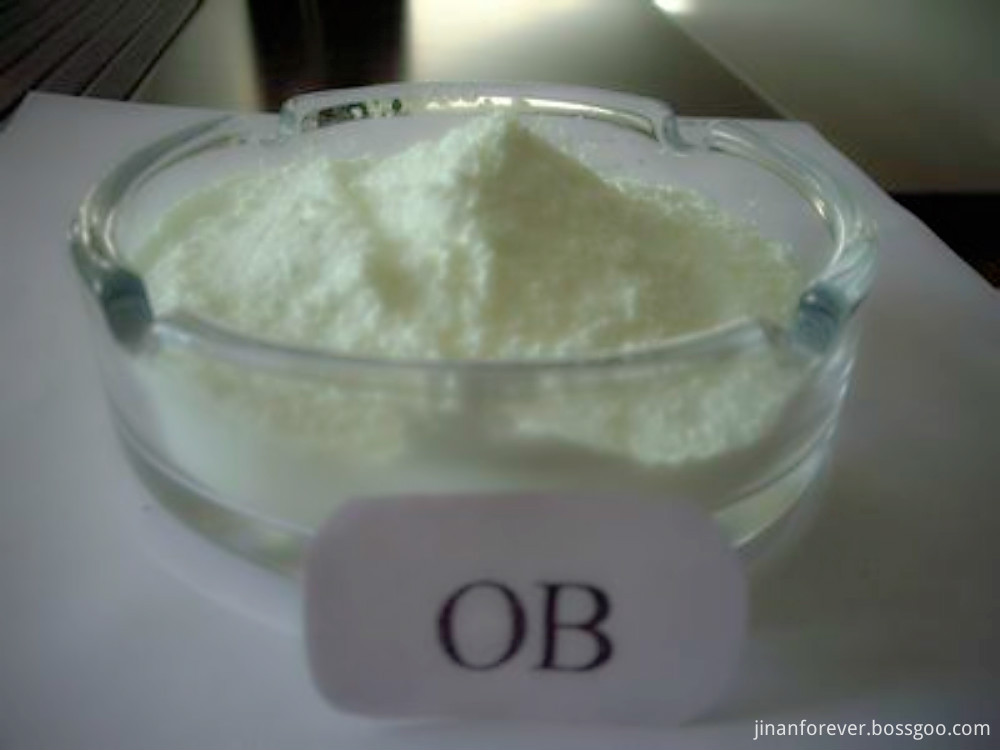# Research on main parameter resonance of a simple harmonic excitation spring force measuring mechanism

Research on main parameter resonance of a simple harmonic excitation spring force measuring mechanism Yang Zhian (Tangshan College of Tangshan Institute of Structural and Vibration Engineering, Tangshan 063000, China) A multi-scale method of nonlinear vibration is used to obtain an approximate solution of the system satisfying the resonance of the main parameters. And numerically calculate it. The effects of excitation, harmonic value and damping on the resonance response curve of the main parameters of the system are analyzed. As the damping decreases and the parameter excitation amplitude increases, the peak and resonance regions of the system's amplitude-frequency response curve increase. When the other parameters are the same, the hard stiffness force amplitude response curve and the soft stiffness force amplitude response curve are antisymmetric with respect to the harmonic adjustment value.

1 Introduction Mechanisms with springs are often used in some measurement control instruments. For example, a spring force measuring mechanism is a mechanism with a spring. When the measured value changes, the position of the mechanism changes correspondingly due to the use of the elastic member, thereby indicating the magnitude of the measured value. However, the frame of such a device is often affected by vibration. Even if various anti-vibration measures are taken, the vibration of the frame will always be transmitted to the meter, which will affect the accuracy of the meter. In addition, the measured value itself is not static, and its fluctuations also affect the indication accuracy of the measuring instrument. The literature gives the series solution of the non-resonant condition of the damper spring force measuring mechanism under the simple harmonic excitation. According to the different positions of the spring force measuring mechanism under the simple harmonic excitation, the analytical solution of the corresponding forced vibration is given. The literature considers the nonlinear factors of the spring force measuring mechanism, and analyzes the main resonance and singularity problems of the spring force measuring mechanism under the simple harmonic excitation. The following is a discussion of the force measuring mechanism shown in Fig. 1 to study the main parameter resonance problem which accounts for the influence of linear and nonlinear factors.

2 The position of the motion differential equation OA of the spring force measuring mechanism is represented by Î±. When the force F on the slider will be at a corresponding position, the force F balances the spring force (spring stiffness k) of the torsion spring acting on the rod OA. If the force F is a periodic disturbance force, for example, F will also vibrate. The rod OA will vibrate around an average position. This average position is called the dynamic equilibrium position and is represented by Î±. If it coincides with the static equilibrium position (indicated by a), the pointer will be error free. However, due to the influence of nonlinearity, Î± and Î± are different, which will bring the dynamic error of the indication. 0. The Lagrangian equation is used to establish the differential equation of motion of the graphic system, and the nonlinear vibration method is used to analyze the dynamic error Yang Zhian. Male, born in November 1963, Qinhuangdao, Hebei, Han nationality. He received his Ph.D. degree in general mechanics from Tianjin University in 1997. He is currently a professor at Tangshan College and a postdoctoral fellow at the School of Mechanical Engineering of Tianjin University. He is mainly engaged in the teaching and research of electromechanical coupling nonlinear dynamics and structure and vibration engineering.

The first draft was received in 20060 and the revised draft was received in 20060530.

difference.

Taking the generalized coordinate as Îµ, which is the swing angle of the rod OA from the dynamic equilibrium position Î±, it is considered that the clockwise direction is positive. Such a system of kinetic energy system's potential energy system dissipation function where Î¼ is the damping coefficient and V is the initial value of the potential energy of the pointer in the vertical position.

The generalized force is the approximate value of F into the Lagrangian equation. After the finishing, the upper formula becomes the first approximate solution and the steady solution of the 3 main parameter resonance. 3.1 Theoretical analysis results due to damping term, nonlinear term and inertial force The term is a small term compared with the linear term. Therefore, the damping parameter and the nonlinear term are preceded by a small parameter Î³. Because the main parameter resonance of the system needs to be studied, the constant term and the forced excitation term are ignored, and the equation (12) becomes Yang Zhi'an: The main parameter resonance study of the simple harmonic excitation spring force measuring mechanism is to substitute equations (14) and (15) into equation (12), and in order to simplify the research process, without loss of generality, in the case of main parameter resonance, Let Ï‰'=1 Î³Ïƒ, where Ïƒ is the tuning value, using the multi-scale method [5~7], taking two time scales T1, and setting the first approximate solution in the case of the main parameter resonance to substituting equation (17) into 16), and using the derivative operator to represent, compare the coefficients of Î³ with the same power to obtain a set of partial differential equations. The solution of equation (18) is where cc represents the conjugate complex part of the right-end function of the equation.

The condition that the formula (20) is substituted into the right end of the formula (19) to eliminate the permanent term is to substitute the formula (21) into the formula (23), and the first approximation solution corresponding to the separation of the real part and the imaginary part is determined to determine the resonance of the corresponding main parameter. The constant solution, let Î² = 0 in equation (24), obtain the square of the algebraic equations and add them to obtain the amplitude Î± and phase Î² algebraic equation or the analytical equation (28). When different solutions are obtained, there are 0 In time, there are c) in other regions only a 3.2 numerical analysis result (28) is the main parameter common amplitude frequency response equation, and the response curve of the main parameter resonance can be calculated by using equation (28), and the response curves of different parameters are analyzed. Impact. If there is no special declaration parameter value, m = can be seen from Figures 2 and 3, the force amplitude response curve is the same whether the hard stiffness system or the soft stiffness system is the same. Figure 4, the rigid stiffness system or the soft stiffness system, as the damping of the mechanism increases, the dynamic error of the pointer, that is, the swing angle of the rod OA from the dynamic equilibrium position Î± is decreasing. At the same time, the resonance range of the pointer is also reduced.

6. As can be seen from Fig. 7, whether the mechanical stiffness is increased with the simple harmonic excitation, the swing angle of the rod OA is also increased, and the resonance range is also expanding. Fig. 8 and Fig. 9 reflect the influence of the tuning value on the amplitude-frequency response curve of the force. It can be seen from Fig. 8 and Fig. 9. Under the same parameters, the amplitude-frequency response curve of the hard stiffness system and the soft stiffness system is about Ïƒ antisymmetry. .

4 Conclusions 1) Establish a nonlinear vibration equation for the damped spring force measuring mechanism subjected to harmonic excitation.

2) Obtain a first approximate solution of the main parameter resonance of the nonlinear vibration equation.

3) Under the same parameters, the force amplitude and frequency response curves of the hard stiffness system and the soft stiffness system are the same.

4) As the damping decreases and the harmonic excitation amplitude increases, the peak and resonance regions of the system amplitude response curve increase.

5) Under the same parameters, the force amplitude frequency response curve of the hard stiffness system and the soft stiffness system is about Ïƒ antisymmetry.

The above conclusions are theoretical analysis and numerical calculation results.

1 Tang Xikuan, Jin Dewen. Institutional Dynamics, Beijing: Higher Education Press, 1984.

Yang Zhian: Research on main parameter resonance of a simple harmonic excitation spring force measuring mechanism 2 Zhu Xiangdong, Yang Zhian. The series solution of the non-resonant condition of the damping force measuring mechanism. Tangshan Teachers 3 Yang Zhian, Zhu Xiangdong. Simple harmonic excitation of forced vibration of an undamped force measuring mechanism. Tangshan College 4 Yang Zhian, Peng Zhen, Cheng Yinghui. The main resonance of the spring-loaded force-measuring mechanism and the Qi 6 Hu Haiyan. Apply nonlinear dynamics. Beijing: Aviation Industry Press, 2000.

Mechanical strength

Since 2005, Forever Chemical has been committed to the research and production of fluorescent whitening agents and their intermediates. We have done a lot of research in the application of Optical Brightener. In the past years, it has provided OEM services for many international famous brands and established long-term cooperative relationships with many famous universities and colleges in China. Optical brightener offers continual quality updates with varied purchasing requirements from our customers.Forever also could give you the best quality in your requirement, competitive price in the market, mature technical support, and professional logistic support. All we want is win-win business. We have the ability to provide our customers with high quality optical brightener, we have the ability to design mature whitening solutions for our customers, we have the ability to tailor our own customized products. We also provide third-party testing and commissioned procurement services for a wide range of international partners.

Generally, in the production process of good water-based ink coatings, the Fluorescent Brightener OB is used. Fluorescent Brightener OB not only increases the whiteness of the ink, but also increases the brightness of the ink. Fluorescent brightener OB-1 improves the grade of the product.

Our commit to quality first, reputation first, customer first, and service first.Fluorescent Brightener OB-1 (FBA 393)

Optical Brightener OB-1,Optical Brighteners Chemistry,Fluorescent Brightening Agent OB-1,Fluorescent Optical Brightener OB-1

Jinan Forever Chemical Co., Ltd. , https://www.jinanforever.com